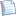# Review 4: Paths in graphsprintable version

R4:     

### Problem R4.1

```def bfs(vertices, source):     for v in vertices: v.dist = INFINITY     source.dist = 0     q = new queue()     q.add(source)     while not q.isEmpty():         u = q.remove()         for v in u.neighbors:             if v.dist == INFINITY:                 v.dist = u.dist + 1                 q.add(v) ```